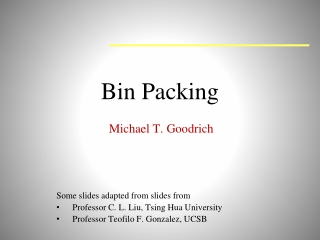DownloadDownload PresentationBin Packing

# Bin Packing

Download Presentation## Bin Packing

- - - - - - - - - - - - - - - - - - - - - - - - - - - E N D - - - - - - - - - - - - - - - - - - - - - - - - - - -
##### Presentation Transcript

1. Bin Packing Michael T. Goodrich Some slides adapted from slides from Professor C. L. Liu, Tsing Hua University Professor Teofilo F. Gonzalez, UCSB

2. .1 .2 .5 .5 .7 .5 .6 .2 .4 Bin Packing Example The bins; (capacity 1) 1 …… .5 .7 .5 .2 .4 .2 .5 .1 .6 Items to be packed

3. Bin Packing Problem Definition • Given n items with sizes s1, s2, ..., sn such that 0 ≤ si ≤ 1 for 1 ≤ i ≤ n, pack them into the fewest number of unit capacity bins. • Problem is NP-hard (NP-Complete for the decision version). • There is no known polynomial time algorithm for its solution, and it is conjectured that none exists.

4. Example Applications Filling recycle bins Loading trucks

5. Historical Application • Mix tapes

6. .1 .2 .5 .5 .7 .5 .6 .2 .4 Bin Packing Optimal Solution Bin Packing Problem 1 …… .5 .7 .5 .2 .4 .2 .5 .1 .6 Optimal Packing MOpt= 4

7. Next-Fit (NF) Algorithm • Check to see if the current item fits in the current bin. If so, then place it there, otherwise start a new bin.

8. .4 .2 .2 .5 .1 .2 .5 .7 .5 .5 .5 .4 .7 .5 .6 .6 .2 Next Fit (NF) Packing Algorithm Example Bin Packing Problem .5 .7 .5 .2 .4 .2 .5 .1 .6 .1 MOpt= 4 Next Fit Packing Algorithm M= 6

9. Approximation Ratios • Approximation Algorithm: • Not an optimal solution, but with some performance ratio guarantee for a given problem instance, I (e.g., no worst than twice the optimal) • Approx. Ratio = Alg(I)/Opt(I)

10. Next Fit (NF) Approximation Ratio • Theorem: Let M be the number of bins required to pack a list I of items optimally. Next Fit will use at most 2M bins. • Proof: • Let s(Bi) be the sum of sizes of the items assigned to bin Bi in the Next Fit solution. • For any two adjacent bins (Bj and Bj+1), we know that s(Bj) + s(Bj+1) > 1.

11. Next Fit (NF) Approximation Ratio • Let k be the number of bins used by Next Fit for list I. We prove the case when k is even (odd case is similar).

12. Next Fit (NF) Lower Bound • There exist sequences such that Next Fit uses 2M −2 bins, where M is the number of bins in an optimal solution. • Proof:

13. First Fit (FF) Algorithm • Scan the bins in order and place the new item in the first bin that is large enough to hold it. A new bin is created only when an item does not fit in the previous bins.

14. .4 .2 .2 .1 .1 .2 .2 .7 .7 .5 .5 .5 .5 .5 .5 .4 .6 .6 First Fit (FF) PackingAlgorithm Example .5 .7 .5 .2 .4 .2 .5 .1 .6 Next Fit Packing Algorithm First Fit Packing Algorithm M= 5

15. Running Time for First Fit • Easily implemented in O(n2) time • Can be implemented in O(n log n) time: • Idea: Use a balanced search tree with height O(log n). • Each node has three values: index of bin, remaining capacity of bin, and best (largest) in all the bins represented by the subtree rooted at the node. • The ordering of the tree is by bin index.

16. Faster First-Fit (FF) Algorithm • 8 bins:

17. First-Fit (FF) Approx. Ratio • Let M be the optimal number of bins required to pack a list I of items. Then First Fit never uses more than ⌈1.7M⌉. • Proof: • [omitted]

18. First-Fit (FF) Approx. Ratio • There exist sequences such that First Fit uses 1.6666…(M) bins. • Proof:

19. First-Fit (FF) Lower Bound • First Fit uses 10M bins, but optimal uses 6M

20. Best Fit Algorithm (BF) • New item is placed in a bin where it fits the tightest. If it does not fit in any bin, then start a new bin. • Can be implemented in O(n log n) time, by using a balanced binary tree storing bins ordered by remaining capacity.

21. Example for Best Fit (BF) • I =  (0.2, 0.5, 0.4, 0.7, 0.1, 0.3, 0.8)

22. Other Heuristics • First Fit Decreasing (FFD): First order the items by size, from largest to smallest, then run the First Fit Algorithm. • Best Fit Decreasing (BFD): First order the items by size, from largest to smallest, then run the Best Fit Algorithm.

23. Experiments • It is difficult to experimentally compute approximation ratios. • It requires that we know the optimal solution to an NP-hard problem! • But we can do experiments for a related parameter: • Define the waste, W(A), for a bin-packing algorithm A to be the number of bins that it uses minus the total size of all n items.

24. Experiments • We are interested in experiments for estimating the waste, W(A), as a function of n and as n grows towards infinity, for random items uniformly distributed in the interval (0,1), for the following algorithms: • A = Next Fit (NF) • A = First Fit (FF) • A = Best Fit (BF) • A = First Fit Decreasing (FFD) • A = Best Fit Decreasing (BFD)